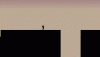# Character movement pauses when shooting its projectile

#### Krisperr

##### MemberMy character has a "skip" in it's movement when they shoot their projectile and I can't figure out what could be causing it. The only culprit I could think of was the "recoil" effect (only used to move the character's hand when the stick is thrown) and removing that hasn't helped any. Unfortunately the mechanic limits a lot of the ideas that I had for level design and I'd love to fix it.

Any help with this one would be greatly appreciated!
Sorry in advance for some of the mess in the code, I've been working on fixing it as I try to fix this issue.

Player Create:

Code:
``````hsp = 0;
vsp = 0;
hsp_frac = 0;
vsp_frac = 0;

hsp_acc = 0.5; //Acceleration
hsp_fric_ground = 0.50;
hsp_fric_air = 0.15;
hsp_walk = 5; // Walk Speed
hsp_wjump = 5; // Wall Jump HSpeed

vsp_jump = -10; // Jump Height
vsp_max = 14; // Max
vsp_wjump = -9; // Wall Jump Height
vsp_max_wall = 7; // Max

onground = false;
onwall = 0;

dust = 0;

grv = 0.5;
grv_wall = 0.1;

jumpbuffer = 0;
walljumpdelay = 0;
walljumpdelay_max = 17;``````
Player Step:

Code:
``````vsp -= vsp_frac;

//Horizontal Collision
///Step Event - where you decide to calculate collisions
if(place_meeting(x+hsp, y, o_wall)){

while(place_meeting(x+hsp, y-y_slope, o_wall) && y_slope <= 2){
y_slope ++;
}

if(place_meeting(x+hsp, y-y_slope, o_wall)){

while(!place_meeting(x+sign(hsp), y, o_wall)){
x+=sign(hsp);
}

hsp = 0;

}else{
y -= y_slope;
}
}

//Horizontal Move
x += hsp;

//Vertical Collision
if (place_meeting(x,y+vsp,o_wall))
{
var onepixel = sign(vsp);
while (!place_meeting(x,y+onepixel,o_wall)) y += onepixel;
vsp = 0;
vsp_frac = 0;
}
//Vertical Move
y += vsp;

//Calc current status
onground = place_meeting(x,y+1,o_wall);
onwall = place_meeting(x+1,y,o_wall) - place_meeting(x-1,y,o_wall);
if (onground) jumpbuffer = 6;

image_speed = 0.8;
if (hsp != 0) image_xscale = sign(hsp);
if (!onground)
{
if (onwall != 0)
{
sprite_index = s_player_wall;
image_xscale = onwall;

var side = bbox_left;
if (onwall == 1) side = bbox_right;
dust++;
if ((dust > 0) && (vsp > 0)) with (instance_create_layer(side,bbox_bottom - 15,"Behind",o_dust))
{
other.dust = 0;
hspeed = -other.onwall*0.5;
}
}
else
{
dust = 0;
sprite_index = s_player_air;
image_speed = 0;
image_index = (vsp > 0);
}
}
else
{
if (hsp != 0) sprite_index = s_player_run;
else sprite_index = s_player;
}``````
Hand Step:

Code:
``````x = o_player.x;
y = o_player.y-4;

image_angle = point_direction(x,y,mouse_x,mouse_y);
image_speed = 0;

if ((mouse_check_button(mb_left))) && (stick_have = true)
{
if (charge < charge_max)
{
sprite_index = s_hand_throw;
image_speed = 0.4;
charge += 2;
}
}

else

if (mouse_check_button_released(mb_left))
{
{
if (charge = charge_max)
{
sprite_index = s_hand;
{
stick_have = false;
recoil = 8;
charge = 0;
with (instance_create_layer(x,y,"Stick",o_stick))
{
speed = 30;
direction = other.image_angle;
image_angle = direction;
}
}
}
}
}

else
{
charge = 0;
}

if (stick_have = true) && (charge = 0)
{
sprite_index = s_hand_stick;
}

recoil = max(0, recoil - 1);

x = x + lengthdir_x(recoil,image_angle);
y = y + lengthdir_y(recoil,image_angle);

if (image_angle > 90) && (image_angle < 270)
{
image_yscale = -1
}
else
{
image_yscale = 1;
}``````
I can't think of any other object that could be causing this, but I can provide any other code needed!

#### Slyddar

##### Member
Place a breakpoint in the hand release code and run in debug mode. Then you can watch the player hsp and see where it's being changed.

#### TheouAegis

##### Member
Where are you setting hsp? Make sure it's not getting set to zero due to a keyboard issue. If you have conflicting keys, maybe hsp is getting set to zero because the direction keys or whatever aren't being read for that one frame.